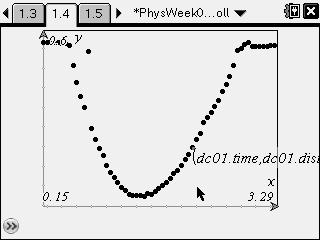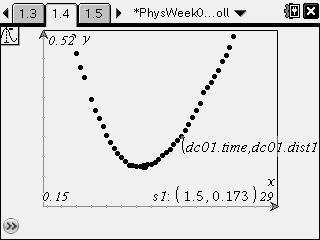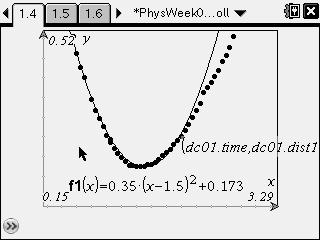Activities

••• Subject Area

• Science: Physics: Kinematics

• Author9-12

45 Minutes

• Device
• TI-Nspire™
• TI-Nspire™ CAS
• Software

TI-Nspire™
TI-Nspire™ CAS

2.1

• Accessories

Sensor - Motion Detector

• Other Materials
• inclined plane at least 1 m long
• small ball, such as a baseball or tennis ball
• safety goggles
• meter stick or tape measure
• Report an Issue

Rolling a Ball on an Inclined Plane

Activity Overview

Students collect distance and time data for a ball rolling up and down an incline.

Key Steps

•Students investigate the motion of a ball rolling up and down an inclined plane.

•Students fit a parabola to the position vs. time graph. They use the characteristics of the data set (i.e., the vertex of the data points) to predict the equation that best fits the data.

•Students then create a tangent to the best-fit curve. They slide the tangent along the curve, recording the slope of the tangent at various points.

Finally, students plot the slope of the tangent line vs. time and find the best-fit equation for the resulting line. They compare this equation to the predicted equation for the velocity of the ball with time.